# CBSE Solutions for Class 10 Maths

#### Select CBSE Solutions for class 10 Subject & Chapters Wise :

If the sum of zeroes of the quadratic polynomial 3x2 – kx + 6 is 3, then find the value of k. (2012)

Here p = 3, q = -k, r = 6
Sum of the zeroes, (α + β) = -q/p= 3 …..(given)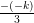= 3
k = 9

If α and β are the zeroes of the polynomial ay2 + by + c, find the value of α2 + β2.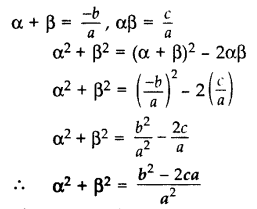If the sum of the zeroes of the polynomial p(x) = (k– 14) x2 – 2x – 12 is 1, then find the value of k. (2017 D)

p(x) = (k2 – 14) x2 – 2x – 12
Here a = k2 – 14, b = -2, c = -12
Sum of the zeroes, (α + β) = 1 …[Given in question]
⇒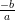= 1
⇒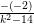= 1
⇒ k2 – 14 = 2
⇒ k2 = 16
⇒ k = ±4

If α and β are the zeroes of a polynomial such that α + β = -6 and αβ = 5, then find the polynomial. (2016 D)

Quadratic polynomial is x2 – (Sum)x + (Product) = 0
⇒ x2 – (-6)x + 5 = 0
⇒ x2 + 6x + 5 = 0

Question 5.
A quadratic polynomial, whose zeroes are -4 and -5, is …. (2016 D)

x2 + 9x + 20 is the required polynomial.

Find the condition that zeroes of polynomial p(x) = ax2 + bx + c are reciprocal of each other. (2017 OD)

Let α and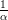be the zeroes of P(x).
P(a) = ax2 + bx + c …(given in the question)
Product of zeroes =⇒ α ×=⇒ 1 =⇒ a = c
Coefficient of x2 = Constant term

Form a quadratic polynomial whose zeroes are 3 + √2 and 3 – √2. (2012)

Sum of zeroes = (3 + √2) + (3 – √2) = 6
Product of zeroes = (3 + √2) x (3 – √2) = (3)2 – (√2)2 = 9 – 2 = 7
Quadratic polynomial = x2 – 6x + 7

Find a quadratic polynomial, the stun and product of whose zeroes are √3 and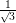respectively. (2014)

Sum of zeroes = √3
Product of zeroes =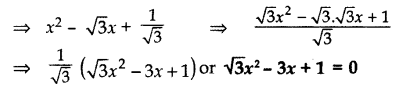Find a quadratic polynomial, the sum and product of whose zeroes are 0 and -√2 respectively. (2015)

x2 – (Sum of zeroes) x + (Product of zeroes)
= x2 – (0)x + (-√2)
= x2 – √2

Divide 4x3 + 2x2 + 5x – 6 by 2x2 + 1 + 3x and verify the division algorithm. (2013)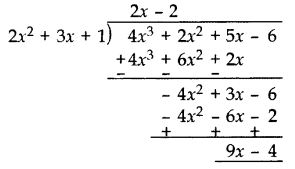Divisor [D]= (2x2 + 3x + 1)

Quotient [Q] = (2x – 2)
Remainder [R] = (9x – 4)
Verification:
[D] × [Q] + [R]
= (2x2 + 3x + 1) × (2x – 2) + 9x – 4
= 4x3 – 4x2 + 6x2 – 6x + 2x – 2 + 9x – 4
= 4x3 + 2x2 + 5x – 6
= Dividend

Given that x – √5 is a factor of the polynomial x3 – 3√5 x2 – 5x + 15√5, find all the zeroes of the polynomial. (2012, 2016)

Let,the polynomial  P(x) = x3 – 3√5 x2 – 5x + 15√5
x – √5 is a factor of the given polynomial.
Put x = -√5,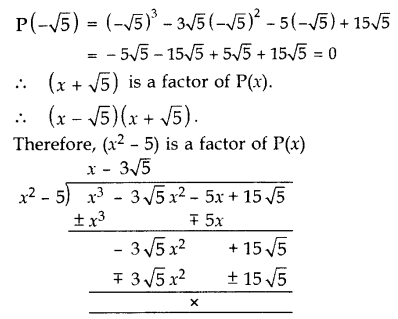Another zero:
x – 3√5 = 0 x = 3√5
All the zeroes of P(x) are -√5, √5 and 3√5.

If a polynomial x4 + 5x3 + 4x2 – 10x – 12 has two zeroes as -2 and -3, then find the other zeroes. (2014)

Given two zeroes are -2 and -3.
(x + 2)(x + 3) = x2 + 3x + 2x + 6 = x2 + 5x + 6
Dividing the given equation with x2 + 5x + 6, we get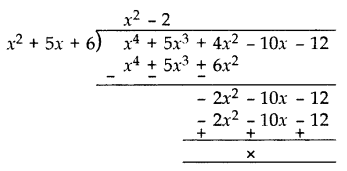x4 + 5x+ 4x2 – 10x – 12
= (x2 + 5x + 6)(x2 – 2)
= (x + 2)(x + 3)(x – √2 )(x + √2 )
Other zeroes are:
x – √2 = 0 or x + √2 = 0
x = √2 or x = -√2

Find all the zeroes of the polynomial 8x4 + 8x3 – 18x2 – 20x – 5, if it is given that two of its zeroes are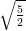and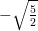. (2014, 2016 D)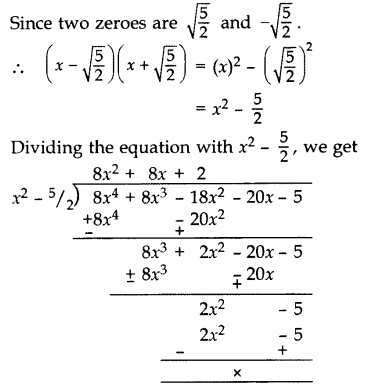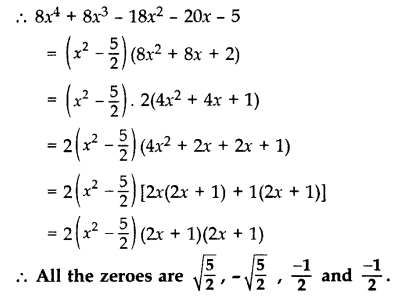If p(x) = x3 – 2x2 + mx + 5 is divided by (x – 2), the remainder is 11. Find m. Hence find all the zeroes of x3 + mx2 + 3x + 1.

p(x) = x3 – 2x2 + mx + 5,
When x – 2,
p(2) = (2)3 – 2(2)2 + m(2) + 5
11 = 8 – 8 + 2m + 5
11 – 5 = 2m
6 = 2m
m = 3
Let q(x) = x3 + mx2 + 3x + 1
= x3 + 3x2 + 3x + 1
= x3 + 1 + 3x2 + 3x
= (x)3 + (1)3 + 3x(x + 1)
= (x + 1)3
= (x + 1) (x + 1) (x + 1) …[ a3 + b3 + 3ab (a + b) = (a + b)3]
All zeroes are:
x + 1 = 0 x = -1
x + 1 = 0 x = -1
x + 1 = 0 x = -1
Hence zeroes are -1, -1 and -1.

Degree of polynomial

 1 x5 +x -25 A 0 2 (x+1)(x3 + x2 + 31) B 2 3 9 C 5 4 x2+1 D 4

1-C, 2-D, 3-A, 4-B

Equations and Root

 1 X2+ 10x + 25 A -5 & 5 2 X2-5x-50 B 5 & 5 3 X2 -25 C -2 & 20 4 X2+18x-40 D -10 & 5

1-B, 2-D, 3-A, 4-C

Equations and Root

 1 X2+ 31x + 30 A -20 & -8 2 X2-28x+160 B -7 & 7 3 X2 -49 C 2 & 3 4 X2+5x+ 6 D 1 & 30

1-D, 2-A, 3-B, 4-C